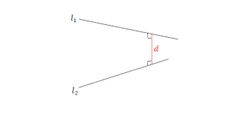## Filters

Q

If the linelies in the plane,

2x−4y+3z=2,

then the shortest distance between this line and the lineis

• Option 1)

2

• Option 2)

1

• Option 3)

0

• Option 4)

3

65 Views

As we have learned

Shortest distance between two skew lines (vector form) -

Shortest distance between is given by

- whereinshortest distance is among the line which is perpendicular to both

shortest distance will be projection of PQ = on LM

So,

Where

So distance  = 0

Option 1)

2

Option 2)

1

Option 3)

0

Option 4)

3

As we have learned

Shortest distance between two skew lines (vector form) -

Shortest distance between is given by

- whereinshortest distance is among the line which is perpendicular to both

shortest distance will be projection of PQ = on LM

So,

Where

So distance  = 0

Option 1)

2

Option 2)

1

Option 3)

0

Option 4)

3

Exams
Articles
Questions